# How to change dataframe column names in PySpark ?

• Last Updated : 15 Feb, 2022

In this article, we are going to see how to change the column names in the pyspark data frame.

Let’s create a Dataframe for demonstration:

## Python3

 `# Importing necessary libraries``from` `pyspark.sql ``import` `SparkSession` `# Create a spark session``spark ``=` `SparkSession.builder.appName(``'pyspark - example join'``).getOrCreate()` `# Create data in dataframe``data ``=` `[((``'Ram'``), ``'1991-04-01'``, ``'M'``, ``3000``),``        ``((``'Mike'``), ``'2000-05-19'``, ``'M'``, ``4000``),``        ``((``'Rohini'``), ``'1978-09-05'``, ``'M'``, ``4000``),``        ``((``'Maria'``), ``'1967-12-01'``, ``'F'``, ``4000``),``        ``((``'Jenis'``), ``'1980-02-17'``, ``'F'``, ``1200``)]` `# Column names in dataframe``columns ``=` `[``"Name"``, ``"DOB"``, ``"Gender"``, ``"salary"``]` `# Create the spark dataframe``df ``=` `spark.createDataFrame(data``=``data,``                           ``schema``=``columns)` `# Print the dataframe``df.show()`

Output :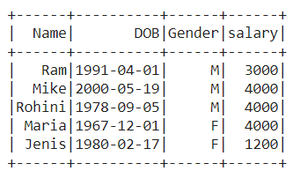### Method 1: Using withColumnRenamed()

We will use of withColumnRenamed() method to change the column names of pyspark data frame.

Syntax: DataFrame.withColumnRenamed(existing, new)

Parameters

• existingstr: Existing column name of data frame to rename.
• newstr: New column name.
• Returns type: Returns a data frame by renaming an existing column.

Example 1: Renaming the single column in the data frame

Here we’re Renaming the column name ‘DOB’ to ‘DateOfBirth’.

## Python3

 `# Rename the column name from DOB to DateOfBirth``# Print the dataframe``df.withColumnRenamed(``"DOB"``,``"DateOfBirth"``).show()`

Output :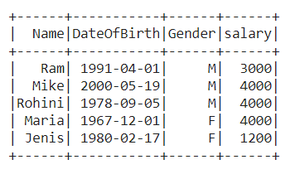Example 2: Renaming multiple column names

## Python3

 `# Rename the column name 'Gender' to 'Sex'``# Then for the returning dataframe``# again rename the 'salary' to 'Amount'``df.withColumnRenamed(``"Gender"``,``"Sex"``).``withColumnRenamed(``"salary"``,``"Amount"``).show()`

Output :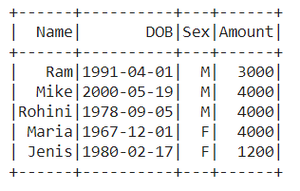### Method 2: Using selectExpr()

Renaming the column names using selectExpr() method

Syntax : DataFrame.selectExpr(expr)

Parameters :

expr : It’s an SQL expression.

Here we are renaming Name as a name.

## Python3

 `# Select the 'Name' as 'name'``# Select remaining with their original name``data ``=` `df.selectExpr(``"Name as name"``,``"DOB"``,``"Gender"``,``"salary"``)` `# Print the dataframe``data.show()`

Output :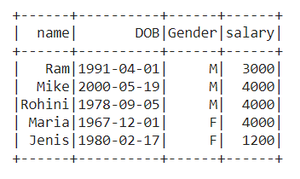### Method 3: Using select() method

Syntax: DataFrame.select(cols)

Parameters :

cols: List of column names as strings.

Return type: Selects the cols in the dataframe and returns a new DataFrame.

Here we Rename the column name ‘salary’ to ‘Amount’

## Python3

 `# Import col method from pyspark.sql.functions``from` `pyspark.sql.functions ``import` `col` `# Select the 'salary' as 'Amount' using aliasing``# Select remaining with their original name``data ``=` `df.select(col(``"Name"``),col(``"DOB"``),``                 ``col(``"Gender"``),``                 ``col(``"salary"``).alias(``'Amount'``))` `# Print the dataframe``data.show()`

Output :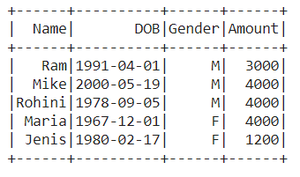### Method 4: Using toDF()

This function returns a new DataFrame that with new specified column names.

Syntax: toDF(*col)

Where, col is a new column name

In this example, we will create an order list of new column names and pass it into toDF function

## Python3

 `Data_list ``=` `[``"Emp Name"``,``"Date of Birth"``,``             ``" Gender-m/f"``,``"Paid salary"``]` `new_df ``=` `df.toDF(``*``Data_list)``new_df.show()`

Output: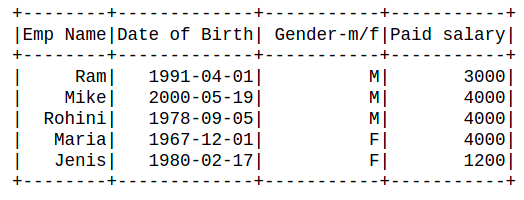My Personal Notes arrow_drop_up